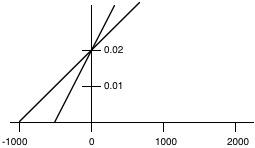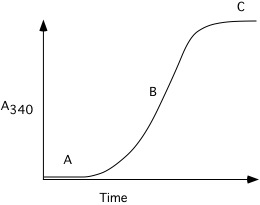# Sample Final Exam

$$\newcommand{\vecs}{\overset { \rightharpoonup} {\mathbf{#1}} }$$ $$\newcommand{\vecd}{\overset{-\!-\!\rightharpoonup}{\vphantom{a}\smash {#1}}}$$$$\newcommand{\id}{\mathrm{id}}$$ $$\newcommand{\Span}{\mathrm{span}}$$ $$\newcommand{\kernel}{\mathrm{null}\,}$$ $$\newcommand{\range}{\mathrm{range}\,}$$ $$\newcommand{\RealPart}{\mathrm{Re}}$$ $$\newcommand{\ImaginaryPart}{\mathrm{Im}}$$ $$\newcommand{\Argument}{\mathrm{Arg}}$$ $$\newcommand{\norm}{\| #1 \|}$$ $$\newcommand{\inner}{\langle #1, #2 \rangle}$$ $$\newcommand{\Span}{\mathrm{span}}$$ $$\newcommand{\id}{\mathrm{id}}$$ $$\newcommand{\Span}{\mathrm{span}}$$ $$\newcommand{\kernel}{\mathrm{null}\,}$$ $$\newcommand{\range}{\mathrm{range}\,}$$ $$\newcommand{\RealPart}{\mathrm{Re}}$$ $$\newcommand{\ImaginaryPart}{\mathrm{Im}}$$ $$\newcommand{\Argument}{\mathrm{Arg}}$$ $$\newcommand{\norm}{\| #1 \|}$$ $$\newcommand{\inner}{\langle #1, #2 \rangle}$$ $$\newcommand{\Span}{\mathrm{span}}$$$$\newcommand{\AA}{\unicode[.8,0]{x212B}}$$

$\mathbf{BioSci\: 102,\\ Sample\: Study\: Problems\: for\: Final\: Exam}$

1. To determine the $$\mathrm{K_{m}}$$ and $$\mathrm{k_{cat}}$$ (= turnover number) of an enzyme, 0.1 nmole of enzyme was added to substrate at various concentrations and the appearance of product was measured.

When the substrate concentration was $$1\times 10^{-5}\: \mathrm{M}$$, the product formed by:

15 seconds was 0.5 $$\mu$$mole

30 seconds was 1.0 $$\mu$$mole

45 seconds was 1.5 $$\mu$$mole

When the substrate concentration was $$2\times 10^{-5}\mathrm{M}$$, the product formed by:

15 seconds was 0.75 $$\mu$$mole

30 seconds was 1.5 $$\mu$$mole

45 seconds was 2.25 $$\mu$$mole

When the substrate concentration was $$4\times 10^{-5}\mathrm{M}$$, the product formed by:

15 seconds was 1.0 $$\mu$$mole

30 seconds was 2.0 $$\mu$$mole

45 seconds was 3.0 $$\mu$$mole

When the substrate concentration was $$10\times 10^{-5}\mathrm{M}$$, the product formed by:

15 seconds was 1.25 $$\mu$$mole

30 seconds was 2.5 $$\mu$$mole

45 seconds was 3.75 $$\mu$$mole

Using the supplied graph paper, and showing all work, calculate the $$\mathrm{K_{m}}$$ and $$\mathrm{K_{cat}}$$ for this enzyme.2. An enzyme was assayed at different concentrations of substrate in the presence and in the absence of an inhibitor. The initial velocities ($$\mathrm{\mu moles\: X\: liter^{-1}\: X\: min^{-1}}$$) were measured at various substrate concentrations (moles/liter) were plotted according to the Lineweaver-Burk method and the following plot was obtained.a) Label the X axis and the Y axis with the appropriate symbols and units.

b) What is the $$\mathrm{K_{m}}$$ of the enzyme for the substrate in the absence of inhibitor?

c) What is the $$\mathrm{V_{max}}$$ of the enzyme solution in the absence of inhibitor?

d) On the graph above, sketch the plot that would be obtained if the amount of inhibitor were decreased to about half the amount originally used.

e) Write an equation which you could be used to determine the $$\mathrm{K_{i}}$$ of the inhibitor from the above information.

3. The $$\mathrm{K_{m}}$$ for an enzyme catalyzed reaction is $$10^{-4}\: \mathrm{M}$$. The apparent $$\mathrm{K_{m}}$$ for the same reaction in the presence of $$9\times 10^{-5}\: \mathrm{M}$$ inhibitor is $$10^{-3}\: \mathrm{M}$$.

a) Is the binding of substrate to enzyme greater or less in the presence of inhibitor? Why?

b) What is the $$\mathrm{K_{i}}$$ for the inhibitor?

c) How would $$\mathrm{V_{max}}$$ without the inhibitor compare with $$\mathrm{V_{max}}$$ with the inhibitor?

4. (12) An enzymatic reaction containing 5 nM enzyme is found to have a $$\mathrm{V_{max}}$$ of 50 nM/min and a $$\mathrm{K_{m}}$$ for substrate of $$mathrm{4\times 10^{-5}\: M}$$. If the enzyme is incubated with substrate at a concentration of $$\mathrm{4\times 10^{-5}\: M}$$ what would be the concentration of the enzyme substrate (ES) complex once the steady state had been reached? Show your reasoning.

5. The enzyme triose-phosphate isomerase catalyzes the interconversion of glyceraldehyde-3-phosphate (G-3-P) and dihydroxyacetone phosphate (DHAP). Which of the following factors influence the rate of this enzyme catalyzed reaction?

$$\mathrm{\Delta G^{\circ'}}$$

[E] or enzyme concentration

$$\mathrm{k_{cat}}$$ or turnover number of the enzyme

T (temperature)

$$\mathrm{K’_{eq}}$$

[G-3-P]

6. Enzyme inhibitors can be classified into four types: Irreversible (I), Competitive (C), Non-competitive (N) and Uncompetitive (U). The following statements are valid for one or more of these four inhibitors. In the space to the left of each statement, indicate by letter (I, C, N, or U) for which inhibitors the statement is true. Indicate all correct answers for each!

Addition of this inhibitor can lead to a decrease in the fraction of enzyme with a bound substrate molecule.

In the presence of this inhibitor, the enzyme's affinity for the substrate appears to have decreased (versus the enzyme's affinity for the substrate in the absence of the inhibitor).

In the presence of this inhibitor, the enzyme's affinity for the substrate is the same as in the inhibitors absence.

This inhibitor can usually be removed from the enzyme by dialysis.

The $$\mathrm{V_{max}}$$ of the enzyme solution is decreased by this inhibitor.

In the presence of this inhibitor the $$\mathrm{K_{m}}$$ of the enzyme for its substrate is larger than the $$\mathrm{K_{m}}$$ value measured for the same substrate in the absence of the inhibitor.

This inhibitor can be bound to the enzyme while the substrate is also bound to the enzyme.

This inhibitor cannot be removed from the enzyme by ion exchange chromatography.

7. The following types of bonds and interactions have been discussed throughout this course.

A) Hydrogen Bond D) Ionic interaction (salt bridge)

B) Hydrophobic interactions E) Peptide bond

C) Disulfide bond F) Glycosidic linkage

For each of the following statements, insert the letter of the one, most appropriate bond or interaction that is being described. (In these statements the word "bond" is used to represent bonds or interactions). A given letter may be used more than once.

The "bond" that is disrupted by urea.

The disruption of these "bonds" results in a change in $$\mathrm{pK_{a}}$$ of hemoglobin after combination with oxygen.

The "bond" responsible for stabilizing the $$\alpha$$-helix structure of proteins.

The type of "bond" broken when asparagine is converted to aspartic acid.

8. One liter of a 0.2 M glycine buffer ($$\mathrm{pK_{a1}=2.34,\: pK_{a2}=9.60}$$) at pH 9.4 is titrated with 0.05 moles of HCl. What is the pH of the resulting solution?

9. Consider the following peptides:

1. his ala pro pro tyr a pentapeptide

2. asn glu trp a tripeptide

3. tyr glu ala arg a tetrapeptide

a) Which would move further toward the anode (+) in electrophoresis at pH 7.0? Why? What would its charge be at pH 7.0?

b) Which would least likely be found in an $$\alpha$$-helix? Why?

c) Draw the structural formula for peptide #3.

d) Which peptide would have the highest isoelectric point? Why?

e) What would the one letter code for the three peptides be?

1)

2)

3)

10. Recently bacteria have been found living in thermal vents (temperatures of $$\mathrm{80^{\circ}C}$$ and above) under the ocean. How might you expect the lipids in the membranes of such bacteria to differ from those of the bacteria in your own gut? Why?

11. The $$\mathrm{\Delta G^{\circ ‘}}$$ of hydrolysis of acetylphosphate to acetate and $$\mathrm{H_{3}PO_{4}}$$ is -10 kcal/mole at pH 7 and $$\mathrm{25^{\circ}C}$$. The $$\mathrm{\Delta G^{\circ ‘}}$$ for hydrolysis of ATP to ADP and Pi is -7.3 kcal/mole under these same conditions.

a) Calculate the $$\mathrm{\Delta G^{\circ ‘}}$$ and $$\mathrm{K_{eq}’}$$ of the following reaction (give units of your answer).b) Assume the concentrations of both ATP and ADP are equal to 3.0 mM in the above reaction mixture and that $$\mathrm{CH_{3}CO_{2}^{-}}$$ is at 5 mM. What concentration of acetylphosphate must be present in order for neither the forward nor the reverse reaction to be thermodynamically favored?

12. For the items below place the symbol "<" (less than), ">" (greater than), "=" (equal) or "?" (cannot say from information given) in the space to indicate the relationship between the two quantities. (e. g. amount of DNA in a human cell > amount of DNA in E. coli cell). Note: MW is abbreviation for molecular weight. (2 pts each).

a) ten H-bonds one covalent bond (e. g. C–C)

b) charge on His at pH 7.0 charge on His at pH 8.0

c) charge on Tyr in solution charge on Asp in the same solution

d) charge on Tyr in solution charge on Asp in a different solution

e) MW of amino acid I MW of Leu

f) MW of Trp MW of amino acid W

g) One hundred H-bonds one covalent bond (e. g. C–C)

h) MW of amino acid E MW of amino acid D

13. A solution of tubulin monomers is warmed to $$\mathrm{37^{\circ}C}$$, and the following change in light scattering (measured by A340) is observed:Explain what is happening at time points "A", "B", and "C".

14. Under one set of conditions the following constants apply to the rate of subunit addition and loss from the two ends of actin filaments:

+ end - end
$$\mathrm{k_{on}\:(\mu M^{-1}S^{-1})}$$ 11.6 1.3
$$\mathrm{k_{off}\: (S^{-1})}$$ 1.4 0.8

Assuming that actin filaments are present in solution at time zero, determine if there will be a net increase or decrease in the length of the filaments over time under the following conditions:

a) 1.0 $$\mu$$M actin monomers

b) 0.5 $$\mu$$M actin monomers

c) 0.1 $$\mu$$M actin monomers

d) Which, if any of the above answers would be changed if all of the actin filaments present at the beginning of the experiment are modified so that no subunit addition or loss can occur from their minus ends?

15. The compound tosyl-phenylalaninechloromethyl ketone (TPCK, below) is an affinity ligand for chymotrypsin.a) (10) Draw the structure of a similar affinity ligand that would be appropriate for trypsin.

b) (8) How would you test the affinity ligand you have drawn to see if it has the desired properties?

16.

a) What forces and interactions are responsible for binding the substrate of chymotrypsin to the active site? Explain.

b) Give an example of a change in the substrate binding site of chymotrypsin (as in a mutation which results in one amino acid being substituted for another) that would alter how tightly the enzyme binds substrate. Indicate whether it would increase or decrease the affinity of the enzyme for substrate.

c) Give an example as in b) except that the mutation would change the activity of the enzyme but not binding. Explain your answer.

Sample Final Exam is shared under a not declared license and was authored, remixed, and/or curated by LibreTexts.